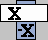## Napier's Bones       for Multiplication, Division & Taking Square Roots

 Contents Vocabularybones / strips / stick multiples of a number References / ResourcesOperationsmultiplydividetake a square rootManipulatives digitalbig papersmaller paperVocabulary     There are 3 kinds of Napier's rods or bones or strips. Digit bones.       More than one digit bone of the same kind is needed to represent a number with more than one of the same digit, 11 and 3345, for example.  Index bone.       It's used to help align the strips and to identify rows on the digit strips.  Square root bone.       Because taking a root requires 3 simultaneous computations, 3 column are needed on the bone. These are the square of the digit, the double of the digit, and the digit.MultiplesEach bone depicts the multiples of the one-digit number at the top of the bone. See the 7 bone above.       Multiples are used here to complete multiplication, division, or taking a root. To obtain multiples of a multi-digit number: 1st: Arrange bones for the multi-digit number to the right of the index bone. 2nd: Recognize each row represents a multiple of that number -- the product of the number and the index number.3rd: Write the sum of the digits in each diagonal of the row, one digit per diagonal.   Carry as needed. This sum is the product, a multiple of the number.Operations       With understanding of multiples and how Napier's Bones make listing of multiples of a number easy, multiplication, division, and the taking of a square root become tasks which may be completed by one who is not great at multiplication.       With attention and repeated multiplication, division, and root taking, one may become more profficient at multiplication.       The following pages and materials are now appropriate. Instructions on the Operations multiplicationdivisionroot extractionManipulatives Napier's Bones Digital ManipulativesNapier's Bones big paper versionNapier's Bones small paper versionMany other manipulatives on many different topics are found at: MathTokens.Com.

 References Azzolino, Agnes "algorithm" © 2005 at www.mathnstuff.com/math/spoken/here/1words/a/a11.htm(last modified 2005), visited 10 April 2009. Azzolino, Agnes "Multiplication & Division" © 2008 at www.mathnstuff.com/math/algebra/tt15.htm#dividevisited 10 April 2009. Azzolino, Agnes "multiple" © 2005 at www.mathnstuff.com/math/spoken/here/1words/m/m29.htmvisited 10 April 2009. Wikipedia®', Wikimedia Foundation, Inc., "Napier's bones - From Wikipedia, the free encyclopedia" GNU Free Documentation License at http://en.wikipedia.org/wiki/Napier%27s_bones(last modified 16 March 2009, at 20:42 (UTC) ), visited 10 April 2009.
 © 2009, October 2010, A. Azzolino www.mathnstuff.com/math/spoken/here/2class/60/nbones.htm